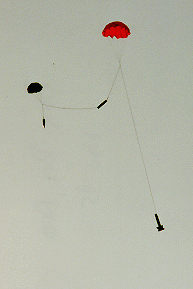#Parachute Descent Calculations

## Problem Statement

How do you determine the right size for your parachute? We can apply an equation from the Rocket Equations Page to figure out the parachute needed for your rocket and how fast your rocket will be going during descent.

The rocket, under its parachute, will speed up toward the ground until the drag force on the chute is equal to the weight of the rocket. So to figure this problem out, we need to find equations for drag force and for the rocket's weight and set them to be equal.

We will try to find the diameter of parachute that brings the rocket down slowly enough that the rocket doesn't break when it hits the ground. How fast is that? It depends on how well you built your rocket, of course, along with where it lands - concrete will hurt more than field grass. If you simply drop your rocket from two feet high, it will hit the ground at a little over 7 mph, or approximately 3 m/s. We'd like to keep the speed to about that.

## Solving the Problem

We will use the drag force, or "wind resistance force" equation, which is

FD = ˝ r Cd A v2

Where
FD is the drag force
r (Greek letter "rho") is the density of air = 1.22 kg/m3
Cd is the drag coefficient
A is the area of the chute
v is the velocity through the air

Meanwhile, the weight of the rocket, otherwise known as the force of gravity (FG), is computed to be

FG = m g

Where
m is the mass of the rocket
g is the acceleration of gravity = 9.81 m/s2

Let's find when they're equal:

FG = FD
m g = ˝ r Cd A v2

...and solving for chute area...

A = (2 m g) / (r Cd v2)

Now the chute area, in terms of the chute diameter, is A = p D2 / 4, so the chute diameter is

D = sqrt(4 A / p).

Combining the two equations above for A & D leads us to the final form of the chute equation as we will use it:

D = sqrt( (8 m g) / (p r Cd v2) )

Where

• D is the chute diameter in meters
• m is the rocket mass in kilograms
• g is the acceleration of gravity = 9.8 m/s2
• p is 3.14159265359
• r is the density of air = 1.22 kg/m3
• Cd is the drag coefficient of the chute, which is 0.75 for a parasheet (flat sheet used for a parachute, like Estes rockets), or 1.5 for a parachute (true dome-shaped chute).
• v is the speed we want at impact with the ground (3 m/s or less)

## Some Examples

### Model Rocket

Let's size a parachute for an Estes Big Bertha.

• m = 62.3 g = 0.0623 kg (from the Estes catalog)
• Cd = 0.75 (since this is a parasheet)
• the rest of the variables are as above, so...

D = sqrt( (8 m g) / (p r Cd v2) ) = sqrt( (8*0.0623*9.81) / (3.14*1.22*0.75*32) ) = 0.435 m

...which is equal to 17 inches. This explains why the Big Bertha comes with an 18 inch chute.

### High-Power Rocket

We'll size a parachute for my LOC V2, which weighs in at 8 pounds even, or 3.6 kg.

• m = 3.6 kg due to all the extra stuff I've added
• Cd = 1.5 because the rocket uses a true domed parachute
• v = 5 m/s I'm increasing v because this rocket's going pretty high and I don't want it to take forever to come down. This is the equivalent to a four-foot drop (ouch).

D = sqrt( (8 m g) / (p r Cd v2) ) = sqrt( (8*3.6*9.81) / (3.14*1.22*1.5*52) ) = 1.4 m

...which is a 4 foot 8 inch parachute. Pretty big. In reality I'm using a RocketMan R7C, which is about that size.

## Finding Descent Velocity

Note that we can easily find the descent velocity, given the chute diameter, by simply rearranging the above equation to get

v = sqrt( (8 m g) / (p r Cd D2) )

## Questions

Your questions and comments regarding this page are welcome. You can e-mail Randy Culp (Tripoli #6926) for inquiries, suggestions, new ideas or just to chat.
Updated 24 August 2008